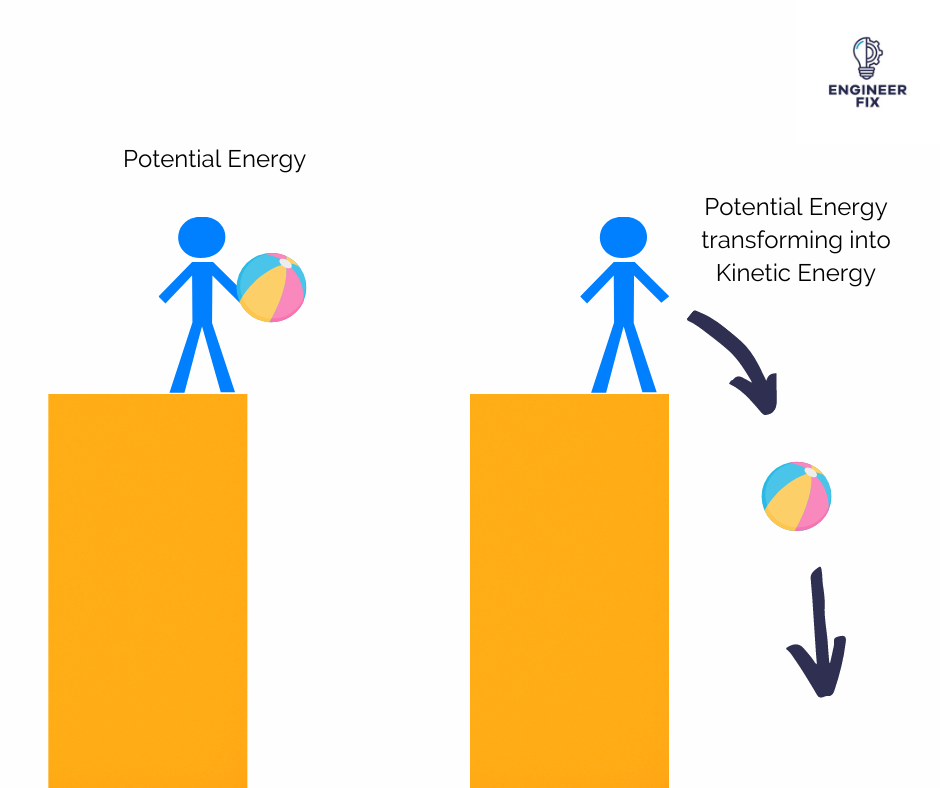# CBSE Class 9 Science Chapter 11 Revision Notes

Chapter 11: Work and Energy Revision Notes

Work Done

• The product of the amount of the force applied on the body and the displacement in the direction of the force is defined as work done on an item. F.s = W
• The work done is 0 if a force acting on a body causes no displacement. For instance, pushing against a wall.

## ENERGY

• The ability to work is defined as energy. It has the same unit of measurement as work.
• Joule (Nm) or Kgm2s2 is the SI unit of energy or work.
• Light, heat, chemical, electrical, and mechanical energy are all examples of energy.

Forms of energy

(i) Kinetic energy (K.E)

(ii) Potential energy (P.E)

Kinetic energy

• The energy of motion is referred to as kinetic energy.
• The energy possessed by a body as a result of its motion, which can occur at any level from the atomic to that of a whole organism.
• This is not an exhaustive list of kinetic energy examples.
• Atomic motion is referred to as electrical motion.
• The movement of waves (electromagnetic or radiant)
• The movement of molecules (thermal or heat)
• Object movement is referred to as motion.
• Sound is the movement of waves through space.
• Thermal/heat energy is commonly referred to as “internal energy” by engineers, while “kinetic energy” is only used in the context of motion.

Potential Energy (Stored energy or gravitational energy)Source:

• The ability to perform labour as a result of one’s position or arrangement.

• As a result of its location or elastic source, an item can store energy.

• At the highest HEIGHT, potential energy is at its highest.

• The conversion of one kind of energy into another is known as energy transformation.

The following are some examples of energy transformation:

• Food is ingested and turned into motion in order to participate in sports or take an exam.

• Radiant – Plants absorb sunlight and transform it into energy for growth.

• Electrical – When energy is supplied to an oven, it is transformed to thermal energy, which is used to heat our meals.

Types of Energy

• There are two types of energy sources: renewable and nonrenewable.
• A renewable energy source is one that is renewed continuously and quickly by natural processes.

The following are some examples of renewable energy sources:

Biomass — The utilisation of a living or formerly alive creature as a source of energy.

Hydropower is the energy generated by the flow of water.

Geothermal – Using heat from deep under the Earth or from the atmosphere near seas to heat homes and other structures.

Wind turbines — wind turbines are used to generate power.

Solar– The use of the sun as a heat source, such as to heat a room within a house, and so on.

Conversion of Energy

• Fossil fuels are a type of energy that comes from the decomposition Chemical, heat, mechanical, and electrical energy are all sources of energy.
• Solar cells are made up of solar cells that absorb sunlight and convert it into electricity.
• When an item is lifted to a greater height, its energy rises.
• The ground level or zero level determines the potential energy of an item at a height.

## POWER

• Work is completed or energy is consumed at a certain rate.
• The fundamental unit of Power is **Watt (P=w/t). **
• One joule of labour per second equals one watt.

Types of Power

• Electrical power is a type of energy that is used to perform tasks.
• Mechanical power is a type of energy that is used to perform tasks (linear, rotary)
• Fluid power is based on the transmission of energy between liquids (hydraulic) and gases (gaseous) (pneumatic)
• The rate at which we consume energy is referred to as power.
• Work or Energy / Time = Power

Source:

]]>